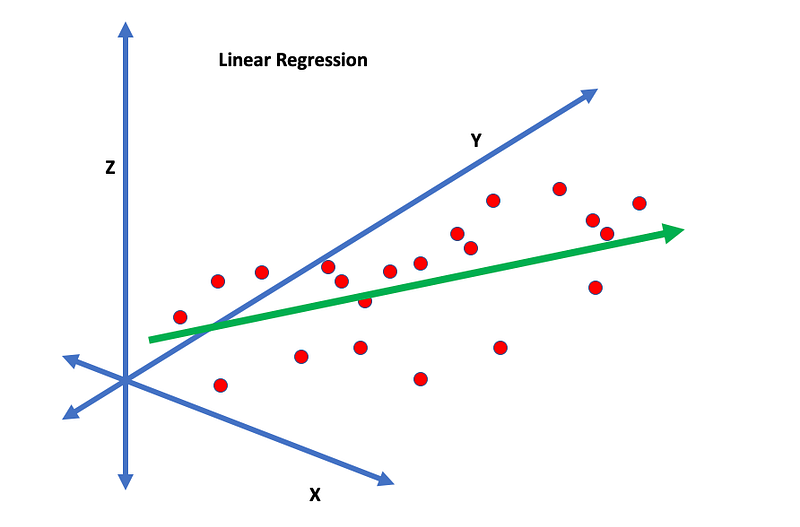# Linear Regression: The Bedrock of Predictive Analytics### Introduction

Predictive analytics stands as a cornerstone of modern data science, influencing decisions across industries — from finance to healthcare, from marketing to operations research. At the heart of predictive analytics lies linear regression, a foundational statistical learning method widely employed in machine learning and data science. This comprehensive article presents a deep dive into the concept of linear regression, exploring its principles, applications, and nuances in predictive analytics.

### Understanding Linear Regression: The Basics

Linear regression is a statistical method used to understand the relationship between two or more variables. At its simplest, linear regression involves two variables, often referred to as the predictor (or independent variable) and the response (or dependent variable). The goal of linear regression is to find a linear relationship or a “best-fit line” between these two variables.

#### Formulation of Linear Regression

Mathematically, this relationship can be represented as:

Y = a + bX + ε

Here,
– Y is the response variable,
– X is the predictor variable,
– a is the Y-intercept (the value of Y when X is zero),
– b is the slope of the line (the change in Y for each one-unit change in X),
– ε is the random error term.

The objective of linear regression analysis is to estimate the values of ‘a’ and ‘b’ that minimize the sum of the squared difference between the actual and predicted values of Y (known as the residuals). This method is known as the method of least squares.

### Types of Linear Regression

Linear regression comes in two primary forms: Simple Linear Regression and Multiple Linear Regression.

#### Simple Linear Regression

In simple linear regression, we predict the response variable Y based on a single predictor variable X. For example, predicting a person’s weight (Y) based on their height (X) would involve simple linear regression.

#### Multiple Linear Regression

Multiple linear regression extends the concept to incorporate more than one predictor variable. In this case, the relationship is represented as:

Y = a + b1*X1 + b2*X2 + … + bn*Xn + ε

This allows for a more complex relationship between the response and predictors, opening up a wider array of applications. For instance, predicting a house’s price based on its size, location, and age would involve multiple linear regression.

### Assumptions in Linear Regression

Linear regression models are built on certain assumptions, which, if violated, can affect the accuracy and reliability of the predictions. These assumptions include:

1. Linearity: There exists a linear relationship between the predictors and the response variable.
2. Independence: Observations are independent of each other.
3. Homoscedasticity: The variance of the residuals is constant across all levels of the predictors.
4. Normality: The residuals of the model are normally distributed.
5. Absence of multicollinearity: In multiple regression, the predictor variables are not highly correlated with each other.

### Applications of Linear Regression

Despite its simplicity, linear regression has wide-ranging applications across various sectors.

– In finance, it can be used to predict future stock prices.
– In healthcare, it can forecast patient outcomes based on different treatment methods.
– In real estate, it can estimate property prices based on different features like location, size, and age.
– In marketing, it can help analyze the effect of advertising spend on sales.

### Linear Regression in Machine Learning

In the realm of machine learning, linear regression is often a starting point for regression tasks. While there are more complex models (like decision trees and neural networks), the simplicity, interpretability, and computational efficiency of linear regression make it a useful tool, especially when the relationship between variables is primarily linear.

### Conclusion

Understanding linear regression is crucial for anyone venturing into data science or machine learning, given its foundational role in these fields. It provides a fundamental statistical approach to understand relationships between variables and make informed predictions. As simple as it may seem, mastering linear regression demands both theoretical understanding and practical application, which form the basis for comprehending more complex machine learning algorithms. As data continues to be the driving force behind decision-making, linear regression will continue to be a critical tool in translating data insights into actionable strategies.

# Personal Career & Learning Guide for Data Analyst, Data Engineer and Data Scientist

## Applied Machine Learning & Data Science Projects and Coding Recipes for Beginners

A list of FREE programming examples together with eTutorials & eBooks @ SETScholars

# Projects and Coding Recipes, eTutorials and eBooks: The best All-in-One resources for Data Analyst, Data Scientist, Machine Learning Engineer and Software Developer

Topics included:Classification, Clustering, Regression, Forecasting, Algorithms, Data Structures, Data Analytics & Data Science, Deep Learning, Machine Learning, Programming Languages and Software Tools & Packages.
(Discount is valid for limited time only)## Find more … …

Demystifying Logistic Regression: A Comprehensive Guide to the Basics, Interpretation, and Applications

Statistics with R for Business Analysts – Linear Regression

Mastering Categorical Variables: Techniques and Best Practices for Predictive Modeling